Open in App
Not now

# Python – Pandas dataframe.append()

• Difficulty Level : Basic
• Last Updated : 11 Jan, 2023

Python is a great language for doing data analysis, primarily because of the fantastic ecosystem of data-centric python packages. Pandas is one of those packages and makes importing and analyzing data much easier. Pandas dataframe.append() function is used to append rows of other dataframe to the end of the given dataframe, returning a new dataframe object. Columns not in the original dataframes are added as new columns and the new cells are populated with NaN value.

Syntax:

`DataFrame.append(other, ignore_index=False, verify_integrity=False, sort=None) `

Parameters:

• other : DataFrame or Series/dict-like object, or list of these
• ignore_index : If True, do not use the index labels.
• verify_integrity : If True, raise ValueError on creating index with duplicates.
• sort : Sort columns if the columns of self and other are not aligned. The default sorting is deprecated and will change to not-sorting in a future version of pandas. Explicitly pass sort=True to silence the warning and sort. Explicitly pass sort=False to silence the warning and not sort.

Return Type: appended : DataFrame

Example #1: Create two data frames and append the second to the first one.

## Python3

 `# Importing pandas as pd``import` `pandas as pd`` ` `# Creating the first Dataframe using dictionary``df1 ``=` `df ``=` `pd.DataFrame({``"a"``:[``1``, ``2``, ``3``, ``4``],``                         ``"b"``:[``5``, ``6``, ``7``, ``8``]})`` ` `# Creating the Second Dataframe using dictionary``df2 ``=` `pd.DataFrame({``"a"``:[``1``, ``2``, ``3``],``                    ``"b"``:[``5``, ``6``, ``7``]})`` ` `# Print df1``print``(df1, ``"\n"``)`` ` `# Print df2``df2`

Output: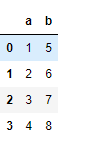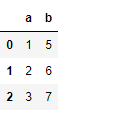Now append df2 at the end of df1.

## Python3

 `# to append df2 at the end of df1 dataframe``df1.append(df2)`

Output: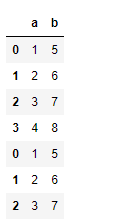Notice the index value of the second data frame is maintained in the appended data frame. If we do not want it to happen then we can set ignore_index=True.

## Python3

 `# A continuous index value will be maintained``# across the rows in the new appended data frame.``df1.append(df2, ignore_index ``=` `True``)`

Output: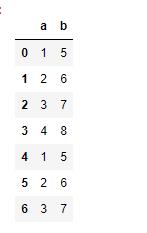Example #2: Append dataframe of different shapes. For unequal no. of columns in the data frame, a non-existent value in one of the dataframe will be filled with NaN values.

## Python3

 `# Importing pandas as pd``import` `pandas as pd`` ` `# Creating the first Dataframe using dictionary``df1 ``=` `pd.DataFrame({``"a"``:[``1``, ``2``, ``3``, ``4``],``                    ``"b"``:[``5``, ``6``, ``7``, ``8``]})`` ` `# Creating the Second Dataframe using dictionary``df2 ``=` `pd.DataFrame({``"a"``:[``1``, ``2``, ``3``],``                    ``"b"``:[``5``, ``6``, ``7``], ``                    ``"c"``:[``1``, ``5``, ``4``]})`` ` `# for appending df2 at the end of df1``df1 ``=` `df1.append(df2, ignore_index ``=` `True``)``df1`

Output: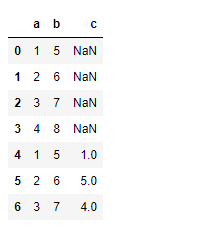Notice, that the new cells are populated with NaN values.

My Personal Notes arrow_drop_up